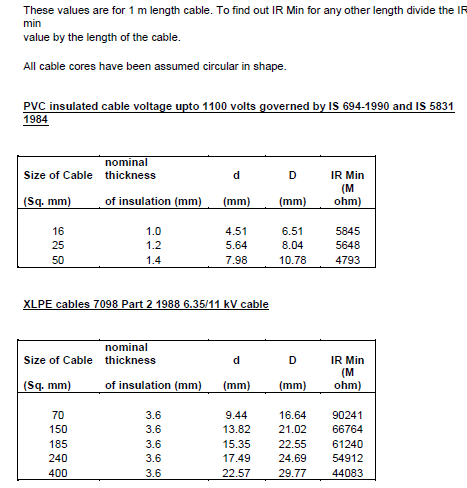## Pages

### Insulation Resistance of Cables

Courtesy: study made by Prof. Shri S K Srivastava, IRIEEN

Insulation resistance is the most commonly measured parameter to check the health of Electrical Insulation. In case of cables the IR differs from cable to cable depending upon the length of cable, insulation thickness and type of cable. For example IR value of 1 meter cable and IR value of 100 meter of same cable are not comparable in absolute term as the later value will be 100th of the former. This paper tries to answer most commonly asked questions such as:

1) What should be the megger voltage?
2) What should be the sample length?
3) What are the limiting values?
4) What is the governing IS?

Insulation Resistance

The Insulation resistance is defined as the Quotient of applied voltage divided by the current measured at a given time from the start of electrification.  We normally measure the insulation resistance by hand driven megger. Initially when we start rotating the instrument, the capacitance across the insulation gets charged due to which the initial current is high thus giving impression of low IR. When the megger is uniformly driven at constant speed for a definite time (1 Min), the voltage applied across the insulation becomes constant and capacitance gets fully charged. The current passing through the insulation gets stabilized to steady state ohmic current and we get constant value of Insulation Resistance.

Voltage and Sample length for IR measurement

If we go through the IS specification for various types of cables i.e. XLPE cable, Elastomeric Cable and PVC insulated cable , we will find that the test method for Insulation resistance for these cables is as per IS 10810 part 43 - 1991 . This IS deals with the measurement of the insulation resistance of the cables. As per above IS the voltage for IR measurement should be 300 ± 30 or 500 ±50 Volt dc.
At this point there is always one question whether HV cables are to be tested by 300 ± 30 or 500 ±50 volt dc IR meter then the answer is yes. If we go through the IS of cables even for HV cable the test method is as per IS 10810 Part 43. And this IS specifies the voltage for the measurement of IR as 300 ± 30 or 500 ±50 volt dc. Thus the voltage rating of cable has no relation to the voltage to be applied for the measurement of IR.
As far as the sample length is concerned as per the relevant IS i.e. IS 10810 Part 43 it should be full drum length or not less than 3 meters.

Limiting Values

Once we are measuring certain parameter to check the condition of insulating material or for that matter for any system then we must know the limiting values. Limiting values means what is the acceptable limit and what is the governing specification.  Since we are discussing here the Insulation resistance parameter for the cable then we must know the limiting values for this and governing specification.

Before going to the parameters some fundamental observations regularly made in the measurement of IR of cable, needs to be discussed.

Let us take a condition where we are carrying out the measurement of two cables of same type and size but with different length say 100 m and 10 m. The measured IR comes same say 5 M ohm then which cable insulation strength is better. The next question comes if one is better then how much better.

Take another condition in which the length of cable is same say 100 m in both the case and type i.e. material of the cable is also same but size is different. In this case also if the measured IR value is same say 5 MOhm then which cable is better.

If we go through the IS for cables we will find that the limiting values for Insulation resistance is not given directly. Instead in these IS specifications the limiting value for Insulation resistance constant or the volume resistivity is given. These parameters take into account the above factors i.e. the size of the cable and the length of cable.

Insulation Resistance constant and Volume resistivity

The insulation resistance constant and volume resistivity parameters are defined as under: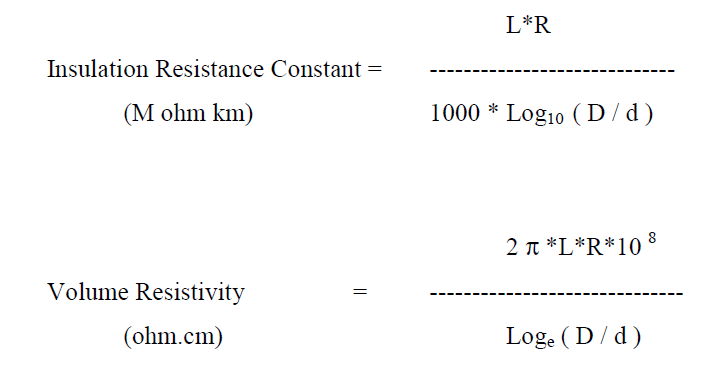Where
R         = Measured Resistance ( M W )
L          = Length of Cable  (m)
D         = Diameter over the insulation excluding screen if any ( mm )
d          = Diameter over the conductor excluding screen if any ( mm )

Incase of shaped conductor D is to be replaced P and d is to be replaced by p where
P          =  Perimeter over the shaped insulated core ( mm )
p          =  Perimeter over the shaped conductor (mm )

Let us take the same condition, which has been discussed in para 3. Two cables of same type and same size but of different length i.e. 100 m and 10 m are showing the same value of Insulation resistance ( 5 M ohm ). The IR constant for these two cases will be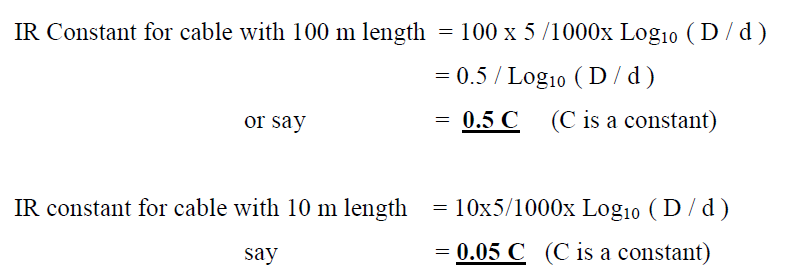We can see here a distinct difference in the health of two cable, which were showing apparently same value of Insulation resistance i.e. 5 M ohm. Similarly we can take the second condition in which the cable size is different but all other parameter like type of cable and length of cable is same. Even if it is showing same value of IR , the IR constant will be different for different size of cables, because of Log10  ( D / d ) factor.  We will be able to differentiate between the health of two cables which apparently showing the same value of IR.

Limiting values as per some IS's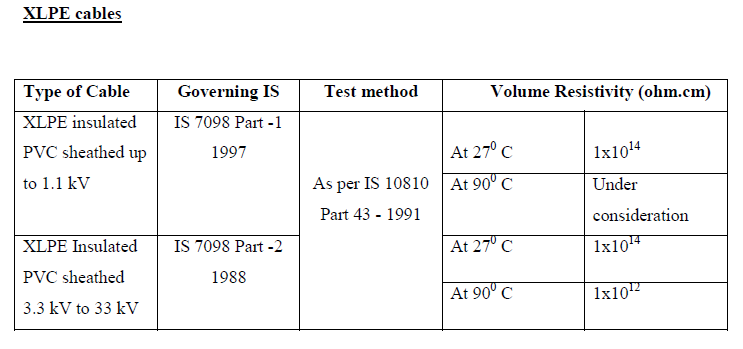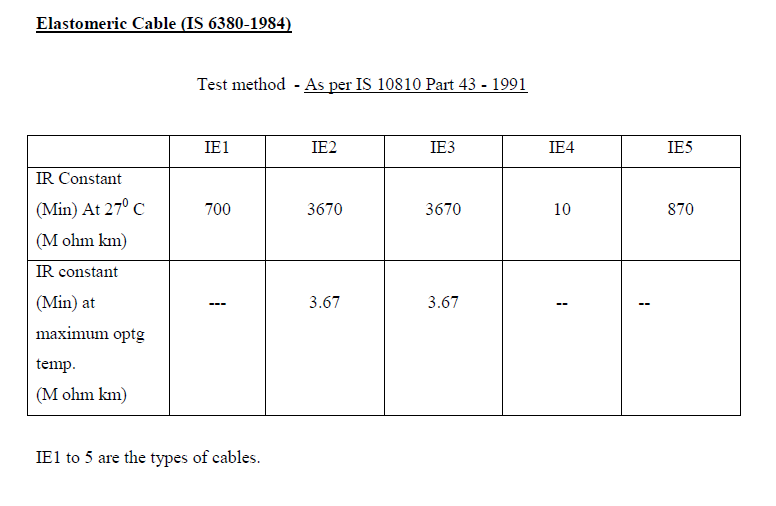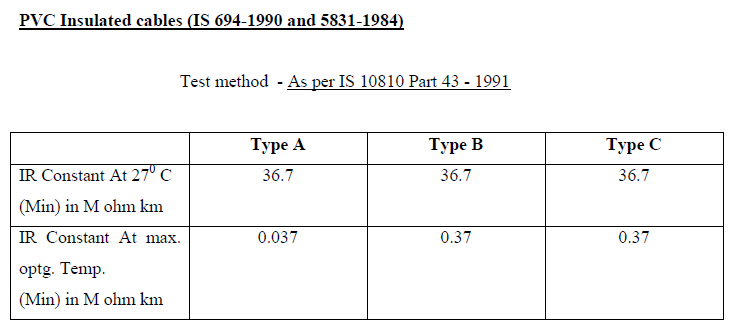Where Type A,B,C are the type of cable.

From the above it can be seen that the minimum value of IR constant or Volume resistivity of the cable is specified in the specification of the cable. Also the test methods for these cable are as per the IS 10810 Part 43 1991.
For better appreciation the IR min value of 1 m length has been calculated (see below) for certain typical cables from the limiting values of IR constant  and volume resistivity as per the corresponding IS. In all these cases circular cable has been assumed and the nominal thickness of insulation has been taken as the insulation thickness.